Kp - the true definition

 Before you read the following, here is a caution. Exam Boards continue to ask on occasions what the units of an equilibrium constant are. You should continue to answer such questions as you have been taught to do, even though, as this article shows, they do not have units.The fault lies in the way that the equilibrium constant is defined at A level.This article also shows how calculations must be performed if the standard pressure used is 100 kPa rather than 1 atm.  Standard pressures and the effect on calculations of Kp. The new A level specifications for 2015 (first assessment 2017) uniformly use a standard pressure of 100 kN m – 2  or 100 kPa in their definition of standard states. This replaces the value of 1 atm which has been used for a very long time. This decision is not without a knock-on effect in thermodynamic calculations, especially on how equilibrium constants should be expressed. Edexcel, OCR, Edexcel and Pre-U specifications all include Kp, the equilibrium constant in terms of partial pressure. The definition of the equilibrium constant for homogeneous gas-phase reactions has been misrepresented in A level for years. It is time that this is recognised and corrected with calculations of the Gibbs function ΔG from equilibrium constants now appearing in A level work. If exam boards are intent on making the standard pressure 100 kN m – 2  or 100 kPa, as has been recommended by IUPAC to replace the previous value of 1 atm,  then the consequence is that the equilibrium constant Kp must be defined correctly. Kp is used for gas mixtures. Equilibrium constants in terms of concentration, Kc , are not affected in quite the same way because the standard state for a solution has not been changed from 1 mol dm – 3. There is a point to be made later about units, however.    Kp: Homogeneous gaseous equilibria. Consider the gaseous equilibrium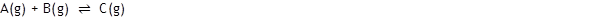The equilibrium constant Kp is generally defined thus: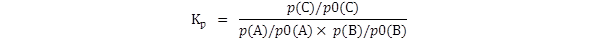where p(A), p(B) and p(C) are the equilibrium partial pressures of the three gases. If this is the expression which is to be used, then the partial pressures must be in atmospheres. If they are not then the numerical value of Kp will be wrong. The definition of  Kp comes from thermodynamic arguments which it is not appropriate to give here. However the values p(A), p(B) and p(C) are not quite what they seem. In the words of a definitive text, Denbigh (1971 - full reference below):  “ It is convenient to choose p0 as unity, in the same units as p.” (Denbigh p.111)  “ The standard pressure p0 has been taken as unity and has not therefore been included explicitly in the equilibrium constant; in fact each p in Kp is really a ratio p/p0 .” (Denbigh p.141)  They are pressures compared with the standard state for a perfect gas, that is of the form p(X)/p0(X) where p0(X) is the standard pressure of the gas. The correct and complete expression for Kp is therefore:It will be immediately clear that if all the p0(X) are 1 atm then there is no numerical effect on the value of Kp. Since each term p(X)/p0(X) is a ratio of two pressures, it is a number. This means that Kp has no units, since all the terms on the right are numbers - which will surprise many of you who have been asked to calculate Kp’s units in examination questions. Kp is a number. The use of 1 atm as the standard pressure simplifies the expression for Kp but has unfortunately led to the belief that it has (or can have) units; initially the 1 atm was omitted but understood, and now is often forgotten. One of the uses of Kp is to calculate the Gibbs free energy change through the expression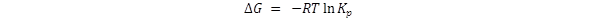The logarithm function operates on numbers only; it does not operate on physical quantities. Therefore Kp must be a number, not some value with units such as atm – 1 . To make Kp a number requires that the standard pressure be included in the Kp expression.   Examples using different values for the standard pressure. Consider the homogeneous gaseous equilibrium already mentioned:1 With a standard pressure of 1 atm. Let us assume that the equilibrium partial pressures are p(A) = 2 atm, p(B) = 3 atm and p(C) = 2 atm. The standard A level text will have it that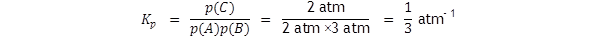But we must include the standard state pressure to get the true equilibrium constant: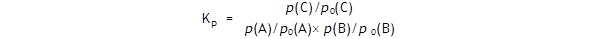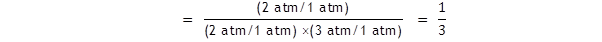This gives the number which we can use to calculate the free energy change G. At 298 K we obtain: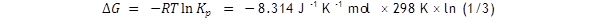The value of ln (1/3) is – 1.1, so ΔG = + 2.72 kJ mol – 1 .   2 With a standard pressure of 100 kN m – 2 or 100 kPa. The equilibrium partial pressures are now, for the same system as above, p(A) = 200 kPa, p(B) = 300 kPa and p(C) = 200 kPa. If you write an expression for Kp without including the standard pressure you will not get the true value ; note the ‘not equal to’ symbol!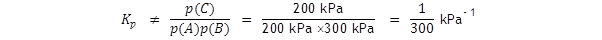This would obviously not give the same value for ΔG as in the first example above. We must include the standard state pressure to get the true equilibrium constant: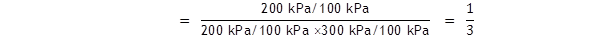This gives the same answers for Kp and ΔG as in example 1. If the standard pressure is to be taken as 100 kPa, as it is in all AS level specifications where the standard state is being discussed, then any calculation of Kp at A2 must involve it if the correct result is to be achieved - unless for this purpose only a standard pressure of 1 atm is adopted.    The equilibrium constant in terms of concentration, Kc The situation is the same here in that the concentrations used in the expression for Kc should all be divided by the standard state concentration, which is 1 mol dm -3. This does not change the numerical value but again it gives a number, as it must do if the logarithm function is to be applied to it.   Reference Denbigh, KG, ‘The Principles of Chemical Equilibrium’. Cambridge University Press, 3rd ed. 1971.